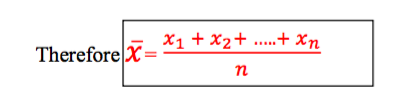# FORM THREE MATHEMATICS STUDY NOTES TOPIC 3-4.

0
865TOPIC 3: STATISTICS

Mean
Calculating the Mean from a Set of Data, Frequency Distribution Tables and Histogram
Calculate the mean from a set of data, frequency distribution tables and histogram
Measures of central tendency:
After
collecting the data, organizing it and illustrating it by means of
diagrams, there is a need to calculate certain statistical measures to
describe the data more precisely. There are various types of measures of
central tendency – the arithmetic mean (or simply the mean), the
median, and the mode. Once the measures of central tendency are found,
it is easier to compare two or more sets of data.
The arithmetic mean
When
people are asked to find the measure of central tendency of some
numbers, they usually find the total of the numbers, and then divide
this total by however many numbers there are. This type of measure of
central tendency is the arithmetic mean. If the n values are x1+x2+x3
………+xn then the arithmetic mean is = x1 + x2+ ….. + xn/nExample 1
The masses of some parcels are 5kg, 8kg, 20kg and 15kg. Find the mean mass of the parcels.
Solution
Total mass = (5 + 8 + 20 + 15) kg = 48kg
The number of parcels = 4
The mean mass = 48kg ÷ 4 = 12kg
The arithmetic mean used as measure of central tendency can be misleading as can be seen in the following example.
Example 2
John
and Mussa played for the local cricket team. In the last six batting
innings, they scored the following number of runs.John: 64, 0, 1, 2, 4,
1;Mussa: 15, 20, 13, 11 , 10, 3.Find the mean score of each player.
Which player would you rather have in your team? Give a reason.
Solution
John’s mean = (64 + 0 + 1 + 2 + 4 + 1) ÷ 6 = 12
Mussa’s mean = (15 + 20 + 13 + 11 + 10 + 3) ÷ 6 = 12
Each
player has the same mean score. However, observing the individual
scores suggests that they are different types of player. If you are
looking for a steady reliable player, you would probably choose Mussa.
Often it is possible to use the mean of one set of numbers to find the mean of another set of related numbers.
Suppose a number a is added to or subtracted from all the data. Then a is added to or subtracted from the mean.
Suppose
the n values are 𝑥!+𝑥! + 𝑥! ………+𝑥!. Multiply each by a, and
we obtain 𝑎𝑥!+𝑎𝑥! + 𝑎𝑥! ………+𝑎𝑥!. So we see that the mean
has been multiplied by a.
Interpreting the Mean Obtained from a Set Data, Frequency Distribution Tables and Histogram
Interpret the mean obtained from a set data, frequency distribution tables and histogram
Measures of central tendency from frequency tables
If the data has already been put into a frequency table, the calculation of the measures of central tendency is slightly easier.
Exercise 1
Juma rolled a six- sided die 50 times. The scores he obtained are summarized in the following table.Calculate the mean score
 Score (x) 1 2 3 4 5 6 Frequency (f) 8 10 7 5 12 8
Solution
10 scores of 2 give a total 10 x 2 = 20
8 scores of 1 gives a total 8 x 1 = 8
And so on, giving a total score of
8 x 1 +10 x 2+7 x 3 + 5 x 4 + 12 x 5 + 8 x 6 = 177
The total frequency = 8 + 10 + 7 + 5 + 12 + 8 = 50
The mean score = 177 ÷ 50 = 3.34